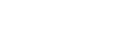## Testing Benton's Law

0

(click on this box to dismiss)

Benford's law, also called the first-digit law, states that in lists of numbers from many (but not all) real-life sources of data, the leading digit is distributed in a specific, non-uniform way. According to this law, the first digit is 1 about 30% of the time, and larger digits occur as the leading digit with lower and lower frequency, to the point where 9 as a first digit occurs less than 5% of the time. This distribution of first digits is the same as the widths of gridlines on the logarithmic scale.Q&A for Ubuntu users and developers

``````-- Testing Benton's Law
-- Benford's law, also called the first-digit law, states that in lists of
-- numbers from many (but not all) real-life sources of data, the leading digit
-- is distributed in a specific, non-uniform way. According to this law, the
-- first digit is 1 about 30% of the time, and larger digits occur as the
-- leading digit with lower and lower frequency, to the point where 9 as a
-- first digit occurs less than 5% of the time. This distribution of first
-- digits is the same as the widths of gridlines on the logarithmic scale.

with data as (Select count(*) as total,
(select max(Reputation) from Users where Reputation > 1) as MAXREP,
(Select cast(Count(*) as decimal) from Users where Reputation > 1 and SUBSTRING(CAST(Reputation AS VARCHAR), 1, 1) = '1') as one,
(Select cast(Count(*) as decimal) from Users where Reputation > 1 and SUBSTRING(CAST(Reputation AS VARCHAR), 1, 1) = '2') as two,
(Select cast(Count(*) as decimal) from Users where Reputation > 1 and SUBSTRING(CAST(Reputation AS VARCHAR), 1, 1) = '3') as three,
(Select cast(Count(*) as decimal) from Users where Reputation > 1 and SUBSTRING(CAST(Reputation AS VARCHAR), 1, 1) = '4') as four,
(Select cast(Count(*) as decimal) from Users where Reputation > 1 and SUBSTRING(CAST(Reputation AS VARCHAR), 1, 1) = '5') as five,
(Select cast(Count(*) as decimal) from Users where Reputation > 1 and SUBSTRING(CAST(Reputation AS VARCHAR), 1, 1) = '6') as six,
(Select cast(Count(*) as decimal) from Users where Reputation > 1 and SUBSTRING(CAST(Reputation AS VARCHAR), 1, 1) = '7') as seven,
(Select cast(Count(*) as decimal) from Users where Reputation > 1 and SUBSTRING(CAST(Reputation AS VARCHAR), 1, 1) = '8') as eight,
(Select cast(Count(*) as decimal) from Users where Reputation > 1 and SUBSTRING(CAST(Reputation AS VARCHAR), 1, 1) = '9') as nine
from Users where Reputation > 1) select
100*one/total as "1",
100*two/total as "2",
100*three/total as "3",
100*four/total as "4",
100*five/total as "5",
100*six/total as "6",
100*seven/total as "7",
100*eight/total as "8",
100*nine/total as "9",
MAXREP,
total
from data;``````

### Enter Parameters

Options:
-Hold tight while we fetch your results
:records returned in :time ms:cached